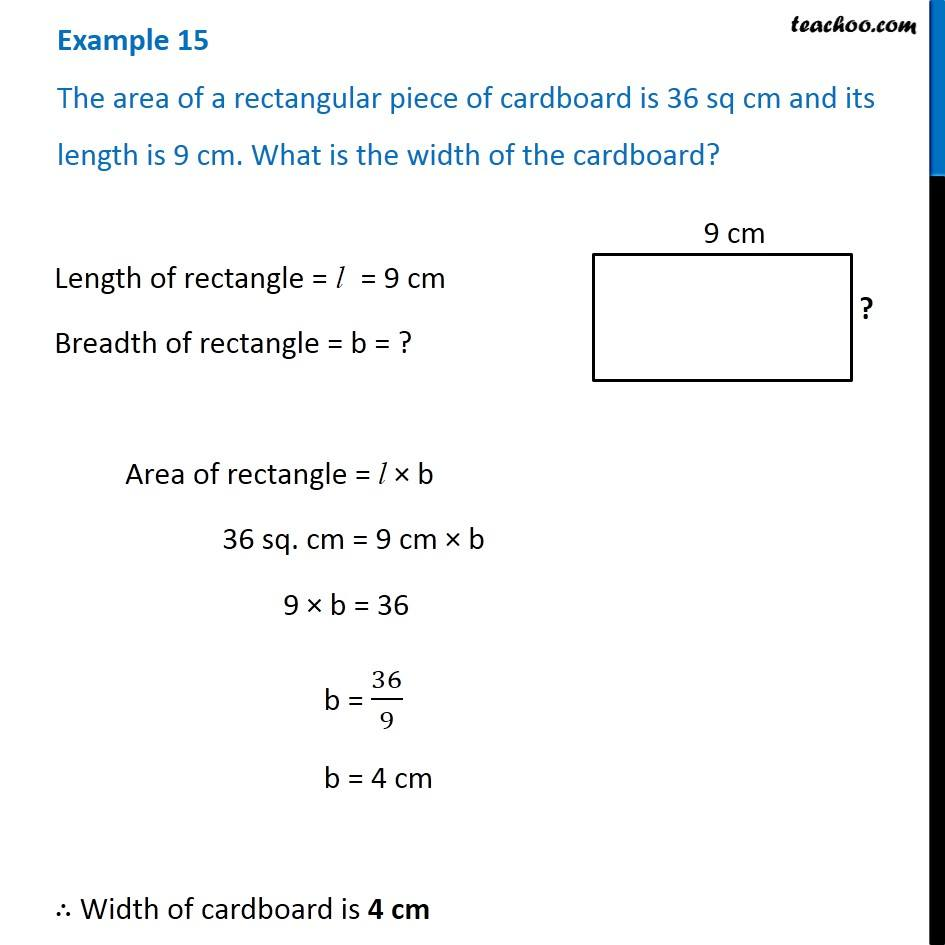Examples

Chapter 10 Class 6 Mensuration
Serial order wiseLearn in your speed, with individual attention - Teachoo Maths 1-on-1 Class

### Transcript

Example 15 The area of a rectangular piece of cardboard is 36 sq cm and its length is 9 cm. What is the width of the cardboard? Length of rectangle = l = 9 cm Breadth of rectangle = b = ? Area of rectangle = l × b 36 sq. cm = 9 cm × b 9 × b = 36 b = 36/9 b = 4 cm ∴ Width of cardboard is 4 cm